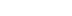/
article

# Simple Intersection Tests For Games

A key component to virtually any graphical game, 2D or 3D, is a collision detection system for game objects. In this article, Looking Glass's Miguel Gomez explains some simple intersection tests for the most useful shapes: spheres and boxes.

Whether it's your car crossing the finish line at 180 miles per hour, or a bullet tearing through the chest of your best friend, all games make use of collision detection for object interaction. This article describes some simple intersection tests for the most useful shapes: spheres and boxes.

Sweep Tests for Moving Objects

A common approach to collision detection is to simply test for whether two objects are overlapping at the end of each frame. The problem with this method is that quickly moving objects can pass through each other without detection. To avoid this problem, their trajectories can be subdivided and the objects checked for overlap at each point; however, this gets expensive if either object experienced a large displacement. On the other hand, a sweep test can efficiently determine a lower and upper bound for the time of overlap, which can then be used as more optimal starting points for the subdivision algorithm.

A Sphere-Plane Sweep Test

Figure 1 shows an example of a quickly moving sphere passing through a plane. It can be seen that C0 is on the positive side of the plane and C1 is on its negative side.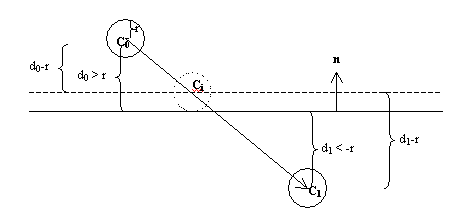Figure 1. A sphere passes through a plane.

In general, if a sphere penetrated a plane at some point during the frame, then d0>r and d1<r, where r is the radius of the sphere and d0 and d1 are the signed distances from the plane to C0 and C1, respectively. The signed distance from a point C to a plane can be calculated with the formula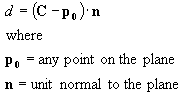More efficiently, we can store the plane in the form {n, D}, where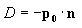The distance d is then calculated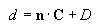The trajectory from C0 to C1 can be parameterized with a variable u, which may be thought of as normalized time, since its value is 0 at C0 and 1 at C1. The normalized time at which the sphere first intersects the plane is given by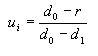The center of the sphere at this time can then be interpolated with an affine combination of C0 and C1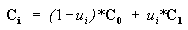This formula interpolates Ci correctly as long as d0 is not equal to d1 (which is the case if displacement has occurred), even when r = 0 (the case of a line segment). If desired, the parameter u can also be used to linearly interpolate the orientation of an object at this point.

In this example, it was assumed that the sphere approached the plane from the positive side and that the sphere was not already penetrating the plane at C0.. In the case that there could have been penetration on the previous frame, the condition |d0|<=r should also be checked. Listing 1 gives an implementation of this sphere-plane sweep test.

Listing 1. A sphere-plane sweep test.

#include "vector.h"

class PLANE

{

public:

VECTOR N;
//unit normal

SCALAR D;
//distance from the plane to the origin from a
//normal and a point

PLANE( const VECTOR& p0, const VECTOR& n ): N(n), D(-N.dot(p0))
{}
//from 3 points

PLANE( const VECTOR& p0, const VECTOR& p1,
const VECTOR& p2 ): N((p1-p0).cross(p2-p0).unit()),
D(-N.dot(p0))
{}
//signed distance from the plane topoint 'p' along
//the unit normal

const SCALAR distanceToPoint( const VECTOR& p ) const
{

return N.dot(p) + D;

}

};

const bool SpherePlaneSweep
(
const VECTOR& C0, //previous position of sphere
const VECTOR& C1, //current position of sphere
const PLANE& plane, //the plane
VECTOR& Ci, //position of sphere when it first touched the plane
SCALAR& u //normalized time of collision

)

{

const SCALAR d0 = plane.distanceToPoint( C0 );
const SCALAR d1 = plane.distanceToPoint( C1 );

//check if it was touching on previous frame
if( fabs(d0) <= r )

{

Ci = C0;
u = 0;
return true;

}

//check if the sphere penetrated during this frame
if( d0>r && d1<r )
{

u = (d0-r)/(d0-d1); //normalized time
Ci = (1-u)*C0 + u*C1; //point of first contact
return true;

}

return false;

}

For the definition of the VECTOR class, please see .

A Sphere-Sphere Sweep Test

Figure 2 shows two spheres that collided between frames. If these spheres experienced acceleration during the frame, their trajectories will be second or higher order curves; however, usually their paths can be accurately approximated as linear segments according to the equations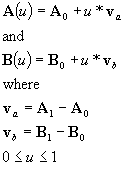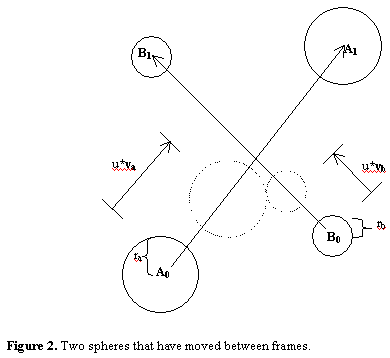Since both spheres traveled for the same amount of time, u is the same for both trajectories. The square of the distance between the lines is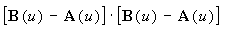and to calculate when they first make contact, we must solve for u such that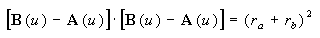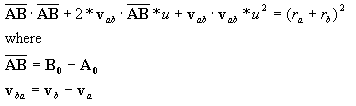The vector vba can be thought of as the displacement of B observed by A. This equation is quadratic in u, so there may be no solution (the spheres never collided), one solution (they just glanced each other), or two solutions (in which case the lesser solution is when they began to overlap and the greater is when they became disjoint again). Again, it is a good idea to check for overlap at the beginning of the frame, since this will handle the case of two stationary spheres. Listing 2 shows an implementation of the sphere-sphere sweep test.

Listing 2. The sphere-sphere sweep test.

#include "vector.h"

template< class T >

inline void SWAP( T& a, T& b )
//swap the values of a and b

{

const T temp = a;
a = b;
b = temp;

}

// Return true if r1 and r2 are real
(
const SCALAR a,
const SCALAR b,
const SCALAR c,
SCALAR& r1, //first
SCALAR& r2 //and second roots
)

{

const SCALAR q = b*b - 4*a*c;
if( q >= 0 )

{

const SCALAR sq = sqrt(q);
const SCALAR d = 1 / (2*a);
r1 = ( -b + sq ) * d;
r2 = ( -b - sq ) * d;
return true;//real roots

}

else

{

return false;//complex roots

}

}

const bool SphereSphereSweep
(
const SCALAR ra, //radius of sphere A
const VECTOR& A0, //previous position of sphere A
const VECTOR& A1, //current position of sphere A
const SCALAR rb, //radius of sphere B
const VECTOR& B0, //previous position of sphere B
const VECTOR& B1, //current position of sphere B
SCALAR& u0, //normalized time of first collision
SCALAR& u1 //normalized time of second collision

)

{

const VECTOR va = A1 - A0;
//vector from A0 to A1

const VECTOR vb = B1 - B0;
//vector from B0 to B1

const VECTOR AB = B0 - A0;
//vector from A0 to B0

const VECTOR vab = vb - va;
//relative velocity (in normalized time)

const SCALAR rab = ra + rb;

const SCALAR a = vab.dot(vab);
//u*u coefficient

const SCALAR b = 2*vab.dot(AB);
//u coefficient

const SCALAR c = AB.dot(AB) - rab*rab;
//constant term

//check if they're currently overlapping
if( AB.dot(AB) <= rab*rab )

{

u0 = 0;
u1 = 0;
return true;

}

//check if they hit each other
// during the frame
if( QuadraticFormula( a, b, c, u0, u1 ) )
{

if( u0 > u1 )
SWAP( u0, u1 );
return true;

}

return false;

}

An Axis-Aligned Bounding Box (AABB) Sweep Test

Just like the name says, the faces of an axis-aligned bounding box are aligned with the coordinate axes of its parent frame (see Figure 3). In most cases AABBs can fit an object more tightly than a sphere, and their overlap test is extremely fast.

To see if A and B overlap, a separating axis test is used along the x, y, and z-axes. If the two boxes are disjoint, then at least one of these will form a separating axis. Figure 4 illustrates an overlap test in one dimension.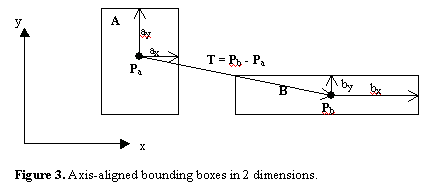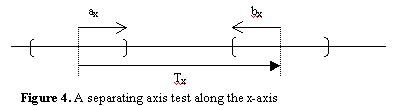In this example the x-axis forms a separating axis because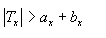Note that the separating axis test will return true even if one box fully contains the other. A more general separating axis test is given in the section below on oriented bounding boxes (OBB’s). Listing 3 defines an AABB class that implements this overlap test.

Listing 3. An AABB class.

#include "vector.h"

// An axis-aligned bounding box

class AABB
{

public:

VECTOR P; //position
VECTOR E; //x,y,z extents

AABB( const VECTOR& p,
const VECTOR& e ): P(p), E(e)

{}

//returns true if this is overlapping b
const bool overlaps( const AABB& b ) const
{

const VECTOR T = b.P - P;//vector from A to B
return fabs(T.x) <= (E.x + b.E.x)

&&

fabs(T.y) <= (E.y + b.E.y)

&&

fabs(T.z) <= (E.z + b.E.z);

}

//NOTE: since the vector indexing operator is not const,
//we must cast away the const of the this pointer in the
//following min() and max() functions
//min x, y, or z

const SCALAR min( long i ) const

{

return ((AABB*)this)->P[i] - ((AABB*)this)->E[i];

}

//max x, y, or z
const SCALAR max( long i ) const

{

return ((AABB*)this)->P[i] + ((AABB*)this)->E[i];

}

};

Just like spheres, AABBs can be swept to find the first and last occurrence of overlap. In Figure 5(a), A and B experienced displacements va and vb, respectively, while Figure 5(b) shows B's displacement as observed by A.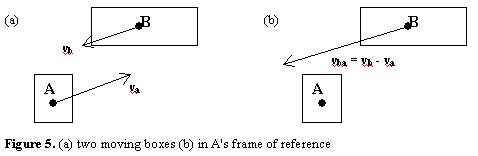Figure 6 shows B's displacement as observed by A.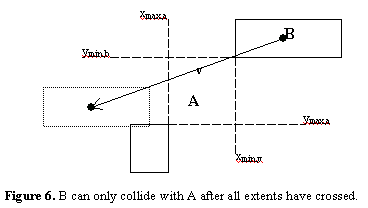In this example, the normalized times it took for the x-extents and y-extents to overlap are given by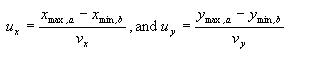and it can be seen that the x-extents will cross before the y-extents. The two boxes cannot overlap until all the extents are overlapping, and the boxes will cease to overlap when any one of these extents becomes disjoint. If u0,x, u0,y, and u0,z were the times at which the x, y, and z-extents began to overlap, then the earliest time at which the boxes could have begun to overlap was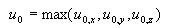Likewise, if u1,x, u1,y, and u1,z are the times at which the x, y, and z-extents become disjoint, then the earliest time at which the boxes could have become disjoint was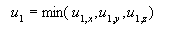In order for the two boxes to have overlapped during their displacement, the condition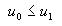must have been met. Just like in the sphere sweep test, the positions of first and last overlap can be linearly interpolated with u. Listing 4 gives an implementation of this AABB sweep algorithm.

Listing 4. An AABB sweep algorithm.

#include "aabb.h"

//Sweep two AABB's to see if and when they first
//and last were overlapping

const bool AABBSweep
(

const VECTOR& Ea, //extents of AABB A
const VECTOR& A0, //its previous position
const VECTOR& A1, //its current position
const VECTOR& Eb, //extents of AABB B
const VECTOR& B0, //its previous position
const VECTOR& B1, //its current position
SCALAR& u0, //normalized time of first collision
SCALAR& u1 //normalized time of second collision

)

{

const AABB A( A0, Ea );//previous state of AABB A
const AABB B( B0, Eb );//previous state of AABB B
const VECTOR va = A1 - A0;//displacement of A
const VECTOR vb = B1 - B0;//displacement of B

//the problem is solved in A's frame of reference

VECTOR v = vb - va;
//relative velocity (in normalized time)

VECTOR u_0(0,0,0);
//first times of overlap along each axis

VECTOR u_1(1,1,1);
//last times of overlap along each axis

//check if they were overlapping
// on the previous frame
if( A.overlaps(B) )
{

u0 = u1 = 0;
return true;

}

//find the possible first and last times
//of overlap along each axis
for( long i=0 ; i<3 ; i++ )
{

if( A.max(i)<B.min(i) && v[i]<0 )
{

u_0[i] = (A.max(i) - B.min(i)) / v[i];

}

else if( B.max(i)<A.min(i) && v[i]>0 )
{

u_0[i] = (A.min(i) - B.max(i)) / v[i];

}

if( B.max(i)>A.min(i) && v[i]<0 )
{

u_1[i] = (A.min(i) - B.max(i)) / v[i];

}

else if( A.max(i)>B.min(i) && v[i]>0 )
{

u_1[i] = (A.max(i) - B.min(i)) / v[i];

}

}

//possible first time of overlap
u0 = MAX( u_0.x, MAX(u_0.y, u_0.z) );

//possible last time of overlap
u1 = MIN( u_1.x, MIN(u_1.y, u_1.z) );

//they could have only collided if
//the first time of overlap occurred
//before the last time of overlap
return u0 <= u1;

}

A Box-Sphere Intersection Test

A very elegant box-sphere intersection test is described in . Figure 7 shows two configurations of a sphere and a box in 2D. Sphere A is closest to an edge, whereas sphere B is closest to a corner. The algorithm calculates the square of the distance from the box to the sphere by analyzing the orientation of the sphere relative to the box in a single loop.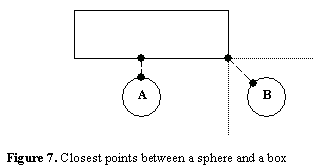If the box is not axis aligned, simply transform the center of the sphere to the box's local coordinate frame. Listing 5 gives an implementation of Arvo's algorithm.

Listing 5. Arvo's algorithm.

#include "aabb.h"

//Check to see if the sphere overlaps the AABB
const bool AABBOverlapsSphere ( const AABB& B, const SCALAR r, VECTOR& C )
{

float s, d = 0;

//find the square of the distance
//from the sphere to the box
for( long i=0 ; i<3 ; i++ )
{

if( C[i] < B.min(i) )
{

s = C[i] - B.min(i);
d += s*s;

}

else if( C[i] > B.max(i) )
{

s = C[i] - B.max(i);
d += s*s;

}

}
return d <= r*r;

}

An Oriented Bounding Box (OBB) Intersection Test

A drawback of using an axis-aligned bounding box is that it can’t fit rotating geometry very tightly.

On the other hand, an oriented bounding box can be rotated with the objects, fitting the geometry with less volume than an AABB. This requires that the orientation of the box must also be specified. Figure 8 shows a 2D example, where A1, A2, B1 and B2 are the local axes of boxes A and B.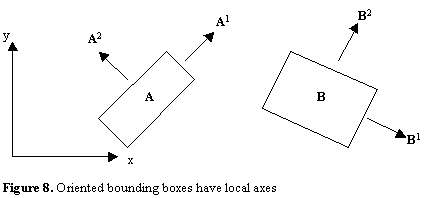For OBBs, the separating axis test must be generalized to three dimensions. A box's scalar projection onto a unit vector L creates an interval along the axis defined by L.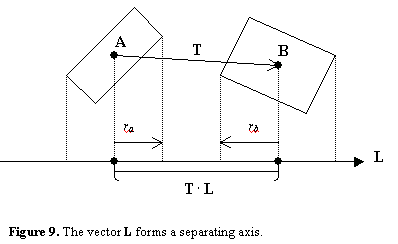The radius of the projection of box A onto L is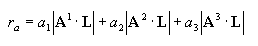The same is true for B, and L forms a separating axis if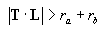Note that L does not have to be a unit vector for this test to work. The boxes A and B are disjoint if none of the 6 principal axes and their 9 cross products form a separating axis. These tests are greatly simplified if T and B’s basis vectors (B1, B2, B3) are transformed into A’s coordinate frame.

An OBB class and an implementation of the OBB overlap test is given in Listing 6 below.

 Listing 6. An OBB class. #include "coordinate_frame.h" class OBB : public COORD_FRAME { public:   VeCTOR E; //extents OBB( const VECTOR& e ): E(e) {} }; //check if two oriented bounding boxes overlap const bool OBBOverlap (   //A VECTOR& a, //extents VECTOR& Pa, //position VECTOR* A, //orthonormal basis //B VECTOR& b, //extents VECTOR& Pb, //position VECTOR* B //orthonormal basis ) {   //translation, in parent frame VECTOR v = Pb - Pa; //translation, in A's frame VECTOR T( v.dot(A), v.dot(A), v.dot(A) ); //B's basis with respect to A's local frame SCALAR R; float ra, rb, t; long i, k; //calculate rotation matrix for( i=0 ; i<3 ; i++ )   for( k=0 ; k<3 ; k++ )   R[i][k] = A[i].dot(B[k]); /*ALGORITHM: Use the separating axis test for all 15 potential separating axes. If a separating axis could not be found, the two boxes overlap. */ //A's basis vectors for( i=0 ; i<3 ; i++ ) {   ra = a[i]; rb = b*fabs(R[i]) + b*fabs(R[i]) + b*fabs(R[i]); t = fabs( T[i] ); if( t > ra + rb ) return false; } //B's basis vectors for( k=0 ; k<3 ; k++ ) {   ra = a*fabs(R[k]) + a*fabs(R[k]) + a*fabs(R[k]); rb = b[k]; t = fabs( T*R[k] + T*R[k] + T*R[k] ); if( t > ra + rb ) return false; } //9 cross products //L = A0 x B0 ra = a*fabs(R) + a*fabs(R); rb = b*fabs(R) + b*fabs(R); t = fabs( T*R - T*R ); if( t > ra + rb ) return false; //L = A0 x B1 ra = a*fabs(R) + a*fabs(R); rb = b*fabs(R) + b*fabs(R); t = fabs( T*R - T*R ); if( t > ra + rb ) return false; //L = A0 x B2 ra = a*fabs(R) + a*fabs(R); rb = b*fabs(R) + b*fabs(R); t = fabs( T*R - T*R ); if( t > ra + rb ) return false; //L = A1 x B0 ra = a*fabs(R) + a*fabs(R); rb = b*fabs(R) + b*fabs(R); t = fabs( T*R - T*R ); if( t > ra + rb ) return false; //L = A1 x B1 ra = a*fabs(R) + a*fabs(R); rb = b*fabs(R) + b*fabs(R); t = fabs( T*R - T*R ); if( t > ra + rb ) return false; //L = A1 x B2 ra = a*fabs(R) + a*fabs(R); rb = b*fabs(R) + b*fabs(R); t = fabs( T*R - T*R ); if( t > ra + rb ) return false; //L = A2 x B0 ra = a*fabs(R) + a*fabs(R); rb = b*fabs(R) + b*fabs(R); t = fabs( T*R - T*R ); if( t > ra + rb ) return false; //L = A2 x B1 ra = a*fabs(R) + a*fabs(R); rb = b *fabs(R) + b*fabs(R); t = fabs( T*R - T*R ); if( t > ra + rb ) return false; //L = A2 x B2 ra = a*fabs(R) + a*fabs(R); rb = b*fabs(R) + b*fabs(R); t = fabs( T*R - T*R ); if( t > ra + rb ) return false; /*no separating axis found, the two boxes overlap */ return true; }

For a more complete discussion of OBBs and the separating axis test, please see . Some other applications of the separating axis test are given next.

An OBB-Line Segment Test

Testing a box and a line segment for intersection requires checking only six separating axes: the box's three principal axes, and the vector cross products of these axes with l, the line direction. Again, the vectors used for these tests do not have to be normalized, and these tests can be simplified by transforming the line segment into the box’s coordinate frame.

One application of this test is to see if a camera's line of sight is obscured. Testing every polygon in a scene could be prohibitively expensive, but if these polygons are stored in an AABB or an OBB tree, a box-segment test can quickly determine a potential set of polygons. A segment-polygon test can then be used to determine if any polygons in this subset are actually obscuring the line of sight.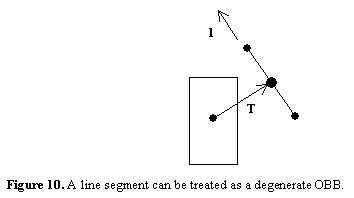The function in Listing 7 assumes the line segment has already been transformed to box space.

Listing 7.

#include "aabb.h"

const bool AABB_LineSegmentOverlap
(

const VECTOR& l, //line direction
const VECTOR& mid, //midpoint of the line
// segment
const SCALAR hl, //segment half-length
const AABB& b //box

)

{

/* ALGORITHM: Use the separating axis
theorem to see if the line segment
and the box overlap. A line
segment is a degenerate OBB. */

const VECTOR T = b.P - mid;
VECTOR v;
SCALAR r;

//do any of the principal axes
//form a separating axis?

if( fabs(T.x) > b.E.x + hl*fabs(l.x) )
return false;

if( fabs(T.y) > b.E.y + hl*fabs(l.y) )
return false;

if( fabs(T.z) > b.E.z + hl*fabs(l.z) )
return false;

/* NOTE: Since the separating axis is
perpendicular to the line in these
last four cases, the line does not
contribute to the projection. */

//l.cross(x-axis)?

r = b.E.y*fabs(l.z) + b.E.z*fabs(l.y);

if( fabs(T.y*l.z - T.z*l.y) > r )
return false;

//l.cross(y-axis)?

r = b.E.x*fabs(l.z) + b.E.z*fabs(l.x);

if( fabs(T.z*l.x - T.x*l.z) > r )
return false;

//l.cross(z-axis)?

r = b.E.x*fabs(l.y) + b.E.y*fabs(l.x);

if( fabs(T.x*l.y - T.y*l.x) > r )
return false;

return true;

}

OBB to AABB conversion

Converting an OBB to an AABB merely involves calculating the extents of the OBB along the x, y, and z-axes of its parent frame. For example the extent of the OBB along the x-axis is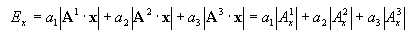The extents along the y and z-axes are calculated similarly.

A Box-Plane Intersection Test

As you can see from Figure 11, a box-plane intersection test only requires checking whether or not n forms a separating axis.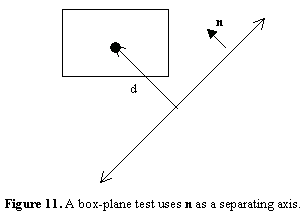The box and the plane overlap if the condition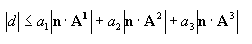is met, where d is the distance from the center of the box to the plane.

Due to time and space, all of the useful intersection tests could not be described here. Some good polygon algorithms are given in  and . References  and  present unique algorithms for generating and manipulating box trees. Bobic and Lander survey the subject of actual collision detection (determining a point of contact and a surface normal) in ,  and , which is definitely an expansive, active area of research. If you plan on employing more advanced collision detection algorithms in your games, you should definitely check out all of the references given below.

Soon after receiving his degree in Physics, Miguel Gomez was lucky enough to land a job as a game programmer. Since then he has programmed physics and graphics for PGA Tour Golf ’96, Hyperblade, Microsoft Baseball 3D, and Destruction Derby 64. He is currently perfecting the collision detection and fluid physics for a kayak racing title at Looking Glass Studios in Redmond. Please send questions and comments to [email protected].

References

 J. Arvo. A simple method for box-sphere intersection testing. In A. Glassner, editor, Graphics Gems, pp. 335-339, Academic Press, Boston, MA, 1990.

 N. Bobic. "Advanced Collision Detection Techniques". Game Developer 6(5):32-42, 1999

 M. Gomez. "C++ Data Structures for Rigid-Body Physics". Gamasutra, July 2, 1999

 S. Gottschalk, M. C. Lin, and D. Manocha. "OBBTree: A Hierarchical Structure for Rapid Interference Detection." In Proc. SIGGRAPH, pp. 171-180, 1996.

 M. Held. "ERIT - A Collection of Efficient and Reliable Intersection Tests". Journal of Graphics Tools, 2(4):25-44, 1997.

 J. Lander. "Crashing into the New Year". Game Developer 6(1):21-27, 1999

 J. Lander. "When Two Hearts Collide". Game Developer 6(2):19-24, 1999

 G. Van den Bergen. "Efficient Collision Detection of Complex Deformable Models Using AABB Trees". Journal of Graphics Tools, 2(4):1-14, 1997.

### Latest Jobs

#### Treyarch

Playa Vista, California
6.20.22
Audio Engineer

#### Digital Extremes

6.20.22
Communications Director

6.20.22
Senior Producer

#### Build a Rocket Boy Games

Edinburgh, Scotland
6.20.22
More Jobs